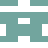### Real Vim ninjas count every keystroke - do you?

###### Pick a challenge, fire up Vim, and show us what you got.

```Your VimGolf key: please sign in

\$ gem install vimgolf
\$ vimgolf setup
\$ vimgolf put 51b4e3b920faf70002000001
```

### Number an outline

The indent and words are right, but the numbers are all wrong.

##### Start file
```1. One
1. One
1. One
1. Two
1. One
1. Two
1. One
1. Three
1. Four
1. Three
1. One
1. One
1. Two
1. Two
1. Four
1. One
1. Two
1. Three
1. Four
1. Five
1. Six
1. Five
1. One
1. Two
1. Three
1. Two
1. One
1. One
1. Two
1. Three
1. Four
1. Five
1. Two
```
##### End file
```1. One
1. One
1. One
2. Two
1. One
2. Two
1. One
3. Three
4. Four
3. Three
1. One
1. One
2. Two
2. Two
4. Four
1. One
2. Two
3. Three
4. Four
5. Five
6. Six
5. Five
1. One
2. Two
3. Three
2. Two
1. One
1. One
2. Two
3. Three
4. Four
5. Five
2. Two
```

```4c4
<   1. Two
---
>   2. Two
6c6
<     1. Two
---
>     2. Two
8,10c8,10
<     1. Three
<     1. Four
<   1. Three
---
>     3. Three
>     4. Four
>   3. Three
13,15c13,15
<       1. Two
<     1. Two
<   1. Four
---
>       2. Two
>     2. Two
>   4. Four
17,22c17,22
<     1. Two
<     1. Three
<     1. Four
<     1. Five
<     1. Six
<   1. Five
---
>     2. Two
>     3. Three
>     4. Four
>     5. Five
>     6. Six
>   5. Five
24,26c24,26
<     1. Two
<     1. Three
< 1. Two
---
>     2. Two
>     3. Three
> 2. Two
29,33c29,33
<     1. Two
<     1. Three
<     1. Four
<     1. Five
<   1. Two
---
>     2. Two
>     3. Three
>     4. Four
>     5. Five
>   2. Two
```

### Solutions by @aaronbieber:

Unlock 5 remaining solutions by signing in and submitting your own entry

## 74 active golfers, 246 entries

##### Solutions by @aaronbieber:28
###### #6 - Aaron Bieber / @aaronbieber

07/14/2013 at 10:05PM30
###### #>10 - Aaron Bieber / @aaronbieber

07/14/2013 at 10:00PM43
###### #>30 - Aaron Bieber / @aaronbieber

07/14/2013 at 09:57PM56
###### #>41 - Aaron Bieber / @aaronbieber

07/14/2013 at 09:53PM72
###### #>67 - Aaron Bieber / @aaronbieber

07/14/2013 at 09:48PM